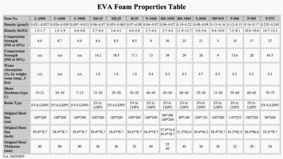### WATER ABSORPTION

Water Absorption, is the amount of water absorbed by a polymer during submersion under pressure.
Calculation:

Calculate the water absorption, expressed in kg/m^2 (lb/ft^2) of cut surfaces (surface without skin or rind) as follows:

Water Absorption = ( W2 - W1 ) / A

where:
W1 = specimen mass before immersion, kg (lb).
W2 = specimen mass after immersion, kg (lb).
A = area of cut surface, m^2 (ft^2).More technical data of EVA foam material